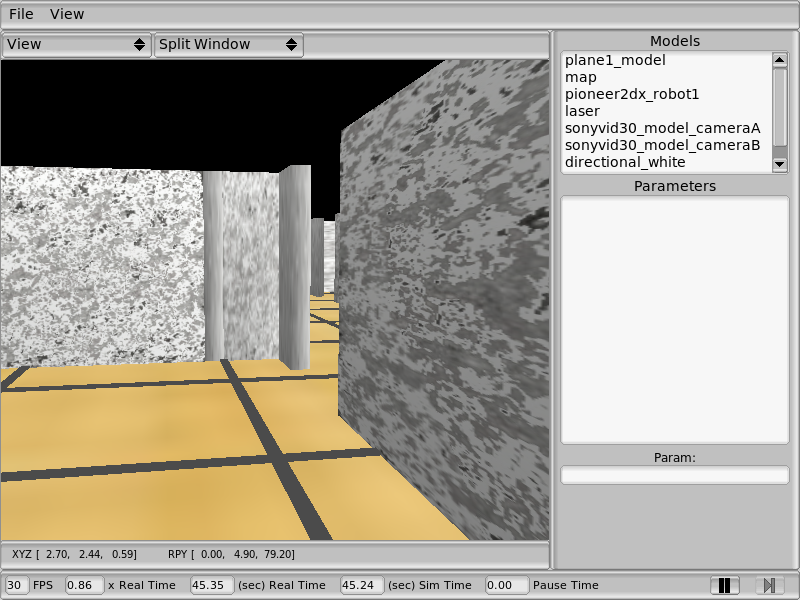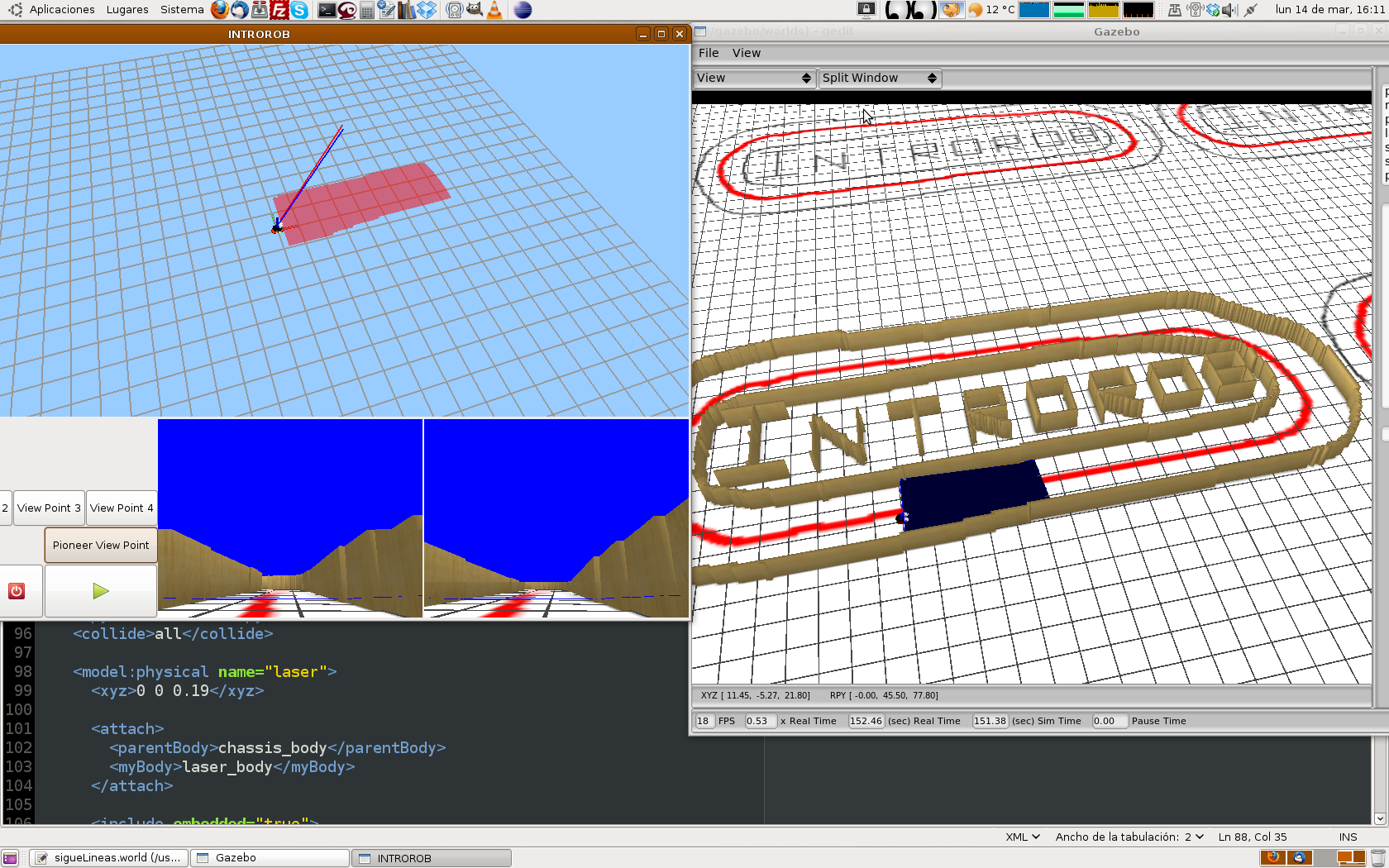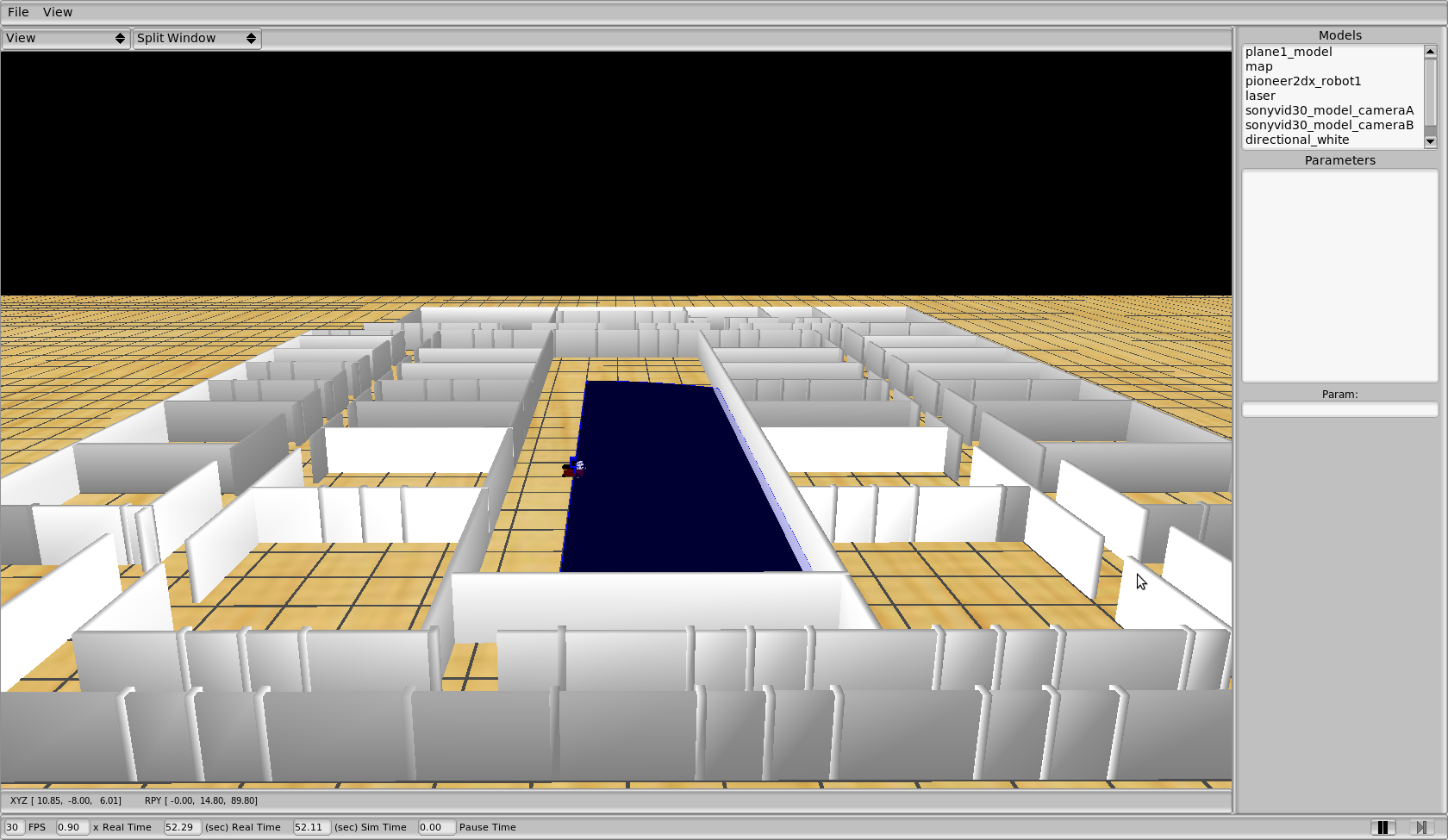## 2011.12.07. Parallelograms attention system

Parallelograms guides the attention system. We can see a blue cross on the floor, which symbolize where the camera is pointing. It has to match with the parallelogram center where camera is pointing to.

Sometimes, the robot attention system generates a random focus-point in order to explore all scene around itself.

## 2011.12.05. Recognizing parallelograms

Now, system is able to hypothesize parallelograms. It just needs to get three points which are forming a parallelogram shape.

## 2011.11.25. Long term memory

In these experiments, the robot goes around the corridor of our building and it does a complete lap.

## 2011.11.23. Geometric model includes Pan & Tilt movements

Now we have included pan & tilt movements to the geometric model.

## 2011.11.22. Short term memory

Here we can see how our system is able to recognize segments from scene around robot.

## 2011.10.11. 3D segments reconstruction with Solis Algorithm

We recognize 2D segments on the 2D image with Solis Algorithm and then, they're back-projected over floor (ground hypothesis). We can see in this image result is plausible.## 2011.10.06. New Visual Memory GUI

Here we can see the new GUI.

## 2011.09.12. Solis Algorithm

We can see the 2D segmentation algorithm based on a Solis' paper. It's work fine, much better than Canny+Hough Transform process.

## 2011.05.26. New GUI with several windows

On this video, we show the new functionality. Our schema includes three windows: main, opengl and navigation controller.

## 2011.05.18. New 3D segments reconstruction with JDE-5.0 implementation

We start to implement our visual memory under Jde-5.0 implementation. Now, our algorithm is inside an Ice component, codified in C++ language.

We're using Gazebo 0.9 as a robotics simulator and a Pioneer 2DX as a robot platform, with two Sony PTZ simulated cameras.

In this first step, we try to recognize 2D segments on the 2D image and then, they're back-projected over floor (ground hypothesis). Our algorithm uses Canny as a border filter and Hough Transform as a segment filter. We can see in this image result is not plausible.## 2011.05.11. Instant 3D segments## 2011.03.17. 3D department with drip effect## 2011.03.14. Follow-line## 2011.03.14. New 3D department building## 2011.03.14. Jarama circuit## 2011.03.11. Introrob class diagram## 2011.02.24. Running new Introrob